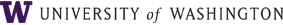Instructor Class Description

Time Schedule:

Laura N Lowes
CEE 457
Seattle Campus

The displacement method in matrix form with programming applications. Fundamentals of modeling of various types of structures. Prerequisite: CEE 377.

Class description

Upon completion of CEE457, students will have the knowledge and skills to use the finite element method to analyze the response of real structures subjected to static loads.

Student learning goals

1. Understand the theory of the finite element method and demonstrate this understanding by a. Formulating the finite element analysis problem for 2D and 3D structures composed of line elements using direct equilibrium, the Principle of Minimum Potential Energy and virtual work. b. Deriving a particular finite element formulation given a displacement field and a constitutive relationship. c. Identifying when the finite element method provides the exact solution and when it provides an approximate solution.

2. Formulating the finite element analysis problem for 2D structures composed of continuum elements using the Principle of Minimum Potential Energy. a. Define the stress and strain state of a 2D continuum as well as the constitutive relationship for a 2D continuum under plane stress or plane strain conditions. b. Derive the finite element formulation for a 2D continuum. c. Use the finite element method to solve 2D continua problems.

3. Solve a global structural analysis problem for an idealized structure, including a. Creating a model of the structure that includes multiple element formulations. b. Writing a Matlab script to solve the global equilibrium equations. c. Evaluating the solution, identifying results that are incorrect or unrealistic, and determining if the solution is exact or approximate.

4. Use a typical commercial structural analysis software package to analyze idealized and real structures. a. Create a model of the real structure that includes i) appropriate material and section properties for concrete, steel and wood, ii) appropriate representation of beams, columns, structural wall elements and slabs using 2D line elements, and iii) appropriate representation of beams, columns, walls and slabs using plate and flat shell elements b. Include in the model representation of a variety of load conditions including point loads, distributed loads, temperature loads and loads associated with initial deformations and imposed displacements. c. Interpret, verify and present the results of a computer-code analysis of a real structure.

General method of instruction

Three 50-minute lectures each week

Recommended preparation

CEE 220, CEE 392 and CEE 379